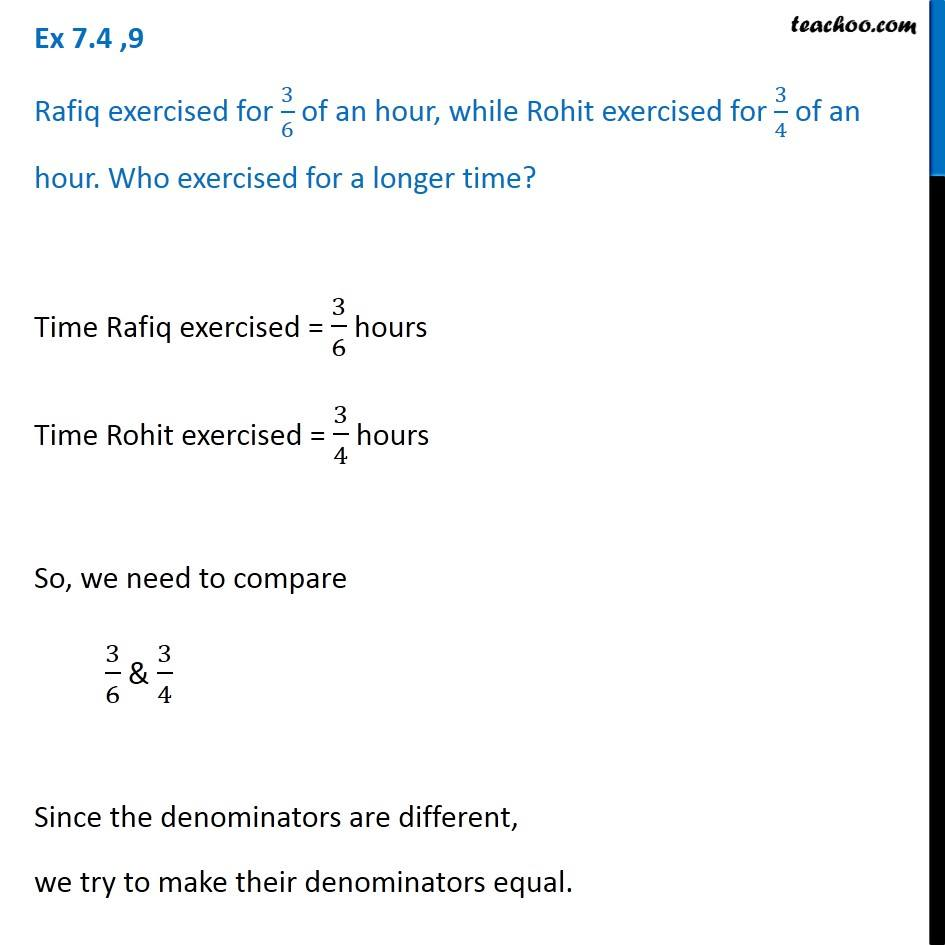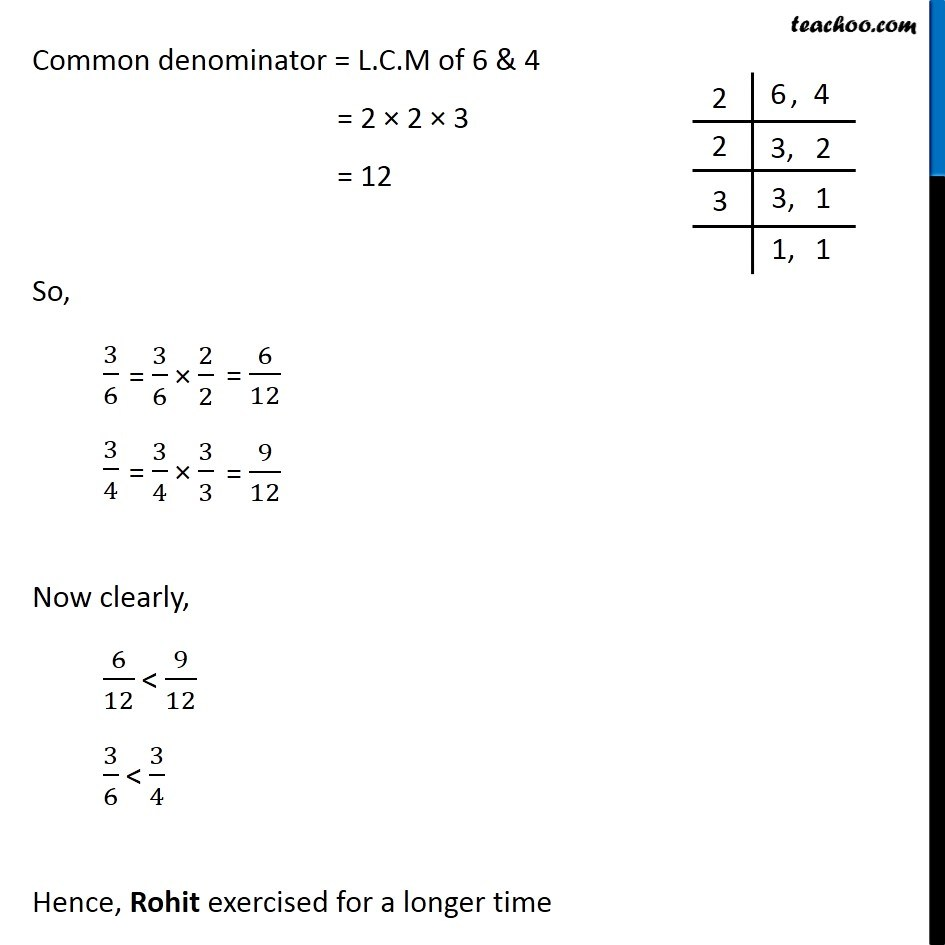Ex 7.4

Chapter 7 Class 6 Fractions
Serial order wiseGet live Maths 1-on-1 Classs - Class 6 to 12

### Transcript

Ex 7.4 ,8 Ila read 25 pages of a book containing 100 pages. Lalita read 2/5 of the same book. Who read less? Ila Total Pages = 100 Pages read = 25 ∴ Fraction read = 25/100 = 25/100 = 5/20 = 1/4 Lalita ∴ Fraction read = 2/5 We need to find who read less. So, we have to find smaller of 1/4 & 2/5 Since the denominator are different, We try to make denominator equal Common denominator = L.C.M of 4 & 5 = 2 × 2 × 5 = 20 So, 1/4 = 2/5 = Since 5/20 < 8/20 ∴ 1/4 < 2/5 Hence, Ila read less of the book